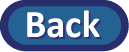### Summary of technology skills learned in this lab:

• Creating numerical and graphical (parallel dotplots or histograms or boxplots) summaries for a quantitative variable.
• Carrying out a "randomization test" simulation to assess the statistical significance of an observed difference in two sample means or two sample medians.

### Summary of statistical skills learned in this lab:

• Review: Interpreting numerical and graphical summaries of a quantitative variable.
• Interpreting a p-value from a computer simulation of a randomization test for a quantitative response variable.
• Interpreting the confidence interval for the difference in two population means.